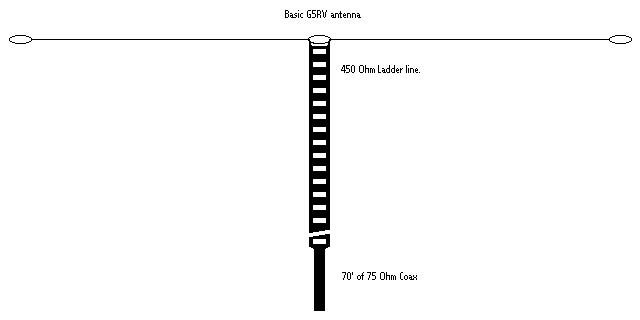G5RV Formula - a simple multiband antenna !

By Rick, KC8AON

Most people think that the famous G5RV antenna was designed as a multi-band antenna, but in fact, it was actually designed as a three - half waves in phase antenna for 14 Mhz by Louis Varney, G5RV. Mr. Varney's version used open wire (600 ohm) feed line all the way to the transmatch instead of the short section of 450 ohm window line and 70 feet of 75 ohm coax that most of us know today.
Mr. Varney also found out that his antenna would work other bands as well with a fair amount of efficiency with the use of the transmatch. As designed, the G5RV exhibits a low SWR on 14 Mhz of around 1.8:1 into a 75 ohm load, but the SWR varies significantly on the other bands, so the transmatch must be used when using it on bands that it was not designed for.

To figure the length of the G5RV antenna as we know it today using the short section of twin lead and coax combo, we simply use 2 simple mathematical formulas. This type of antenna can be designed for any frequency, so the conventional dimensions will be different for different bands.
To figure the "flat top" (dipole) length, we use the formula: 1428 / F Mhz = Length in feet, and the formula of the matching is: 468 / F Mhz X velocity of the twin lead = Length in feet.So, to figure a conventional G5RV, we devide 1428 by 14 Mhz, which equals 102 feet. This is the total length of the flat top section, and since it is fed at the center, this makes each half 51 feet long.
Now for the matching section, we will use 450 ohm window line with a velocity factor of 98% for the equations, so, 468 devided by 14 Mhz equals 33.43 feet, multiplied by 98% = 32.76 feet . So we now have a 102 foot dipole fed at the center with a 32.76 foot long section of 450 ohm window line, now just connect 70 feet of 75 ohm coax between the 450 ohm line and tuner, and you are ready to go on the air.

To demonstrate the difference in size for different bands, we will now figure one for 15 meters as an example. So, 1428 devided by 21 Mhz = 68 feet, this is the flat top, so we devide it by 2 to find the center and this makes each side 34 feet long. For the matching section, we devide 468 by 21 Mhz = 22.29 feet multiplied by 98% = 21.84 feet of 450 ohm window line, we now attach 70 feet of 75 ohm coax and have a fair match on 15 meters.
Notice that the 15 meter version is almost the same size as a conventional coax fed dipole which is about 66 feet long, so this 15 meter antenna will work very well from 40 meters on up when using a transmatch. Of couse, you can do as Mr. Varney did and run the 450 ohm window line all the way to the balanced output of your tuner eliminating even more loss and giving your efficiency a big boost!

The G5RV, is not the answer to everyones antenna problems, but when space limitations dictate a compromise, it sure can save the day! And remember, when you have space limitations, there is absolutely no need to be stuck on one band - you simply learn to improvise !73...........KC8AON